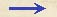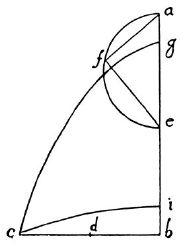Discorsi Propositions 3/11-pr-04 Discorsi Proposition3/11-pr-04PROBLEMA, PROPOSITIO XI. PROBLEM. PROPOSITION XIDato impetu et amplitudine semiparabolae, altitudinem eius reperire. Given the amplitude and the speed [impetus] at the terminal point of a semi-parabola, to find its altitude. {30} Impetus datus definitus sit a perpendiculo ad horizontem ab; amplitudo vero in horizontali sit bc: oportet, sublimitatem semiparabolae reperire, {300} cuius impetus sit ab, amplitudo vero bc. Constat ex iam demonstratis, dimidiam amplitudinem bc futuram esse mediam proportionalem inter altitudinem et sublimitatem ipsius semiparabolae, cuius impetus ex praecedenti, est idem cum impetu cadentis ex quiete in a per totam ab; est propterea ba ita secanda, ut rectangulum a partibus eius contentum aequale sit quadrato dimidiae bc, quae sit bd. Hinc apparet, necessarium esse quod db dimidiam ba non superet: rectangulorum enim a partibus contentorum maximum est, cum tota linea in partes {10} secatur aequales. Dividatur itaque ba bifariam in e: quod si ipsa bd aequalis fuerit be, absolutum est opus, eritque semiparabolae altitudo be, sublimitas vero ea (et ecce parabolae elevationis semirectae amplitudinem, et supra demonstratum est, omnium esse maximam ab eodem impetu descriptarum). At minor sit bd quam dimidia ba, quae ita seconda est, ut rectangulum sub partibus quadrato bd sit aequale. Supra ea semicirculus describatur, in quo ex a applicetur af, aequalis bd, et iungatur fe, cui secetur pars aequalis eg: erit iam rectangulum bga cum quadrato eg aequale quadrato ea, cui quoque {20} aequalia sunt duo quadrata af, fe. Demptis itaque quadratis ge, fe aequalibus, remanet rectangulum bga aequale quadrato af, nempe bd, et linea bd media proportionalis inter bg, ga: ex quo patet, semiparabolae cuius amplitudo bc, impetus vero ab, altitudinem esse bg, sublimitatem ga. Quod si ponatur inferius bi aequalis ga, erit haec altitudo, ia vero sublimitas semiparabolae ic. Let the given speed be represented by the vertical line ab, and the amplitude by the horizontal line bc; it is required to find the sublimity of the semi-parabola whose terminal speed is ab and amplitude bc. (Condition 3/05-pr-02-cor) From what precedes [Cor. Prop. V] it is clear that half the amplitude bc is a mean proportional between {300} the altitude and sublimity of the parabola of which the terminal speed is equal, in accordance with the preceding proposition, to the speed acquired by body in falling from rest at a through the distance ab. Therefore the line ba must be cut at a point such that the rectangle formed by its two parts will be equal to the square of half bc, namely bd. Necessarily, therefore, bd must not exceed the half of ba; for of all the rectangles formed by parts of a straight line the one of greatest area is obtained when the line is divided into two equal parts. Let e be the middle point of the line ab; and now if bd be equal to be the problem is solved; (Condition 3/10-th-07) for be will be the altitude and ea the sublimity of the parabola. Incidentally we may observe a consequence already demonstrated, namely: of all parabolas described with any given terminal speed that for which the elevation is 45° will have the maximum amplitude.) But suppose that bd is less than half of ba which is to be divided in such a way that the rectangle upon its parts may be equal to the square of bd. Upon ea as diameter describe a semicircle efa, in which draw the chord af, equal to bd: join fe and lay off the distance eg equal to fe. Then the rectangle bg.ga plus the square of eg will be equal to the square of ea, and hence also to the sum of the squares of af and fe. If now we subtract the equal squares off e and ge there remains the rectangle bg.ga equal to the square of af, that is, of bd, a line which is a mean proportional between bg and ga; (Condition 3/05-pr-02-cor) from which it is evident that the semi-parabola whose amplitude is bc (Condition 3/10-th-07) and whose terminal speed [impetus] is represented by ba has an altitude bg and a sublimity ga. If however we lay off bi equal to ga, (Condition 3/05-pr-02-cor) then bi will be the altitude of the semi-parabola ic, and ia will be its sublimity.Discorsi Propositions 3/11-pr-04 Discorsi Proposition3/11-pr-04